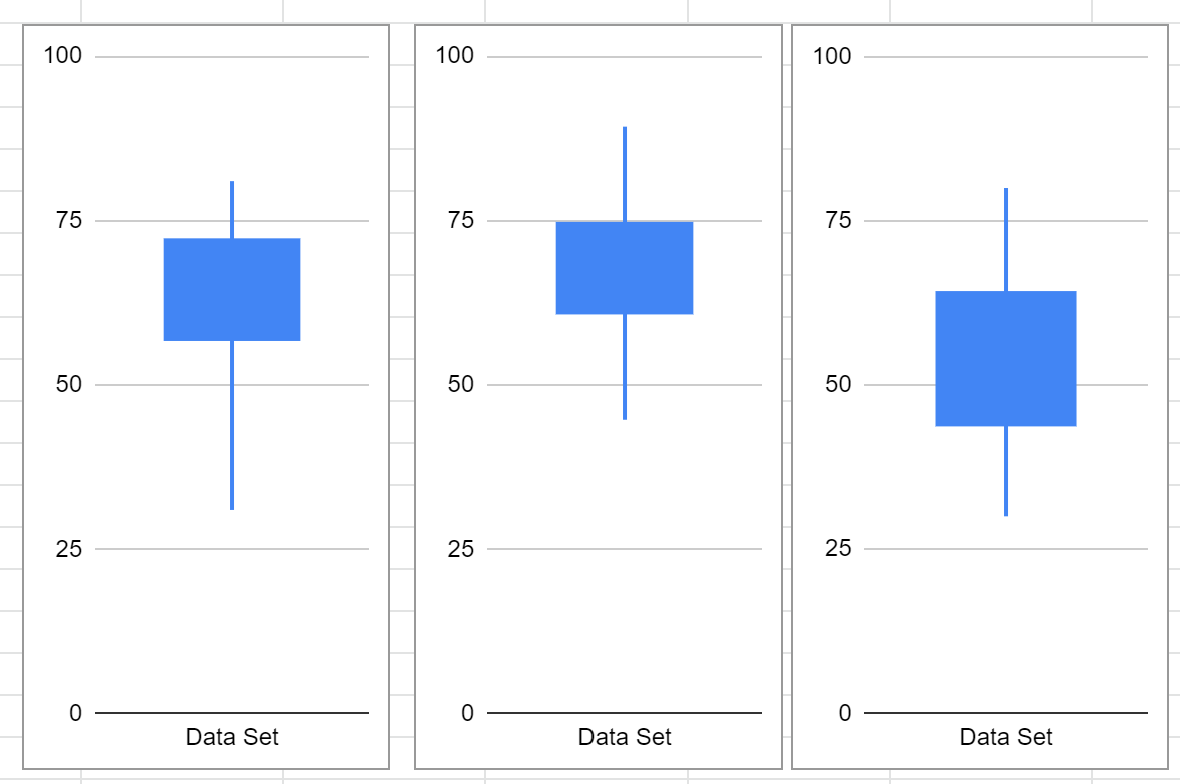# Data Analysis - Box & Whisker

A Box and Whisker chart can be a useful way to represent a set of marks.The box represents the range covered by the middle half of the marks (quartile 2 and 3). The whiskers represent quartiles 1 and 4 (the bottom and top quarters). At a glance you can see the range, as well as where most marks are.

Here’s how to create such a graph in Google Sheets.

1. Have your set of marks in column A with a heading. In this example the marks go from A2 to A44.
2. In B1 have the formula: =min(A2:A44)
3. C1: =quartile(A2:A44,1)
4. D1: =quartile(A2:A44,3)
5. E1: =max(A2:A44)
6. Click on cell A1, then go to Insert Chart and under Chart Type choose Candlestick Chart.